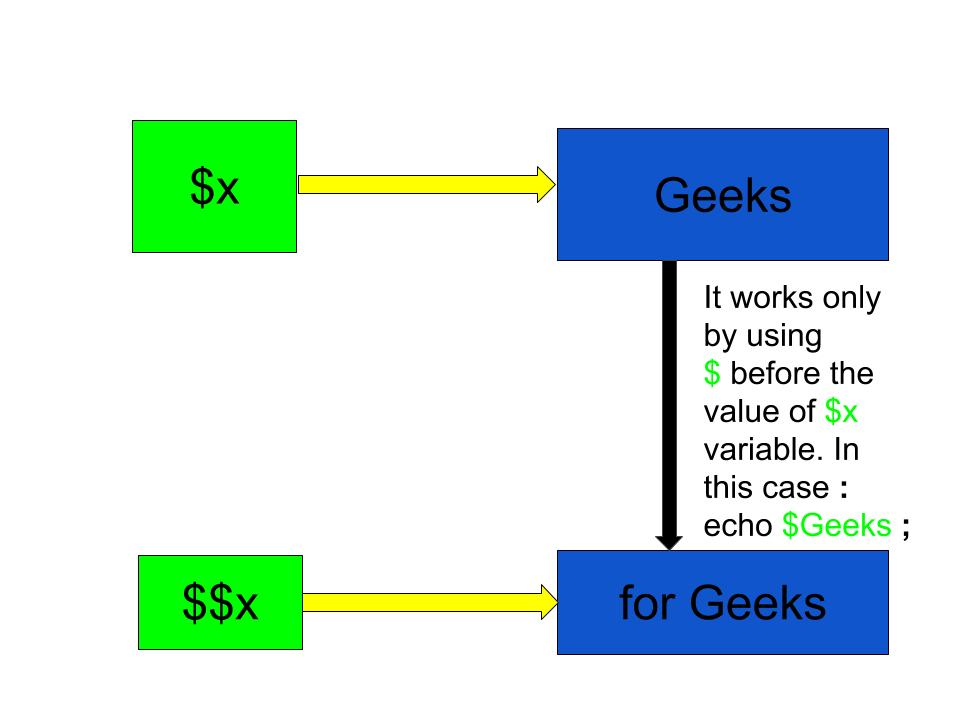# What does \$\$ (dollar dollar or double dollar) means in PHP ?

• Last Updated : 21 Jun, 2022

The \$x (single dollar) is the normal variable with the name x that stores any value like string, integer, float, etc. The \$\$x (double dollar) is a reference variable that stores the value which can be accessed by using the \$ symbol before the \$x value. These are called variable variables in PHP.

Variable Variables:- Variable variables are simply variables whose names are dynamically created by another variable’s value.

From the given figure below, it can be easily understood that:

• \$x stores the value “Geeks” of String type.
• Now reference variable \$\$x stores the value “for Geeks” in it of String type.So, the value of “for geeks” can be accessed in two ways which are listed below:

• By using the Reference variable directly. Example: echo \$\$x;
• By using the value stored at variable \$x as a variable name for accessing the “for Geeks” value. Example: echo \$Geeks; which will give output as “for Geeks” (without quote marks).

Examples:

```Input : \$x = "Geeks";
\$\$x = for Geeks;
echo "\$x ";
echo "\$\$x;";
echo \$Geeks;
Output : Geeks
for Geeks
for Geeks

Input : \$x = "Rajnish";
\$\$x = "Noida";
echo "\$x lives in " . \$Rajnish;
Output : Rajnish lives in Noida```

Below are examples illustrating the use of \$ and \$\$ in PHP:

Example-1:

## php

 ``

Output:

```Geeks
GeeksforGeeks
GeeksforGeeks```

Example-2:

## php

 ``

Output:

```Geeks
GeeksforGeeks
computer science
computer science```

My Personal Notes arrow_drop_up